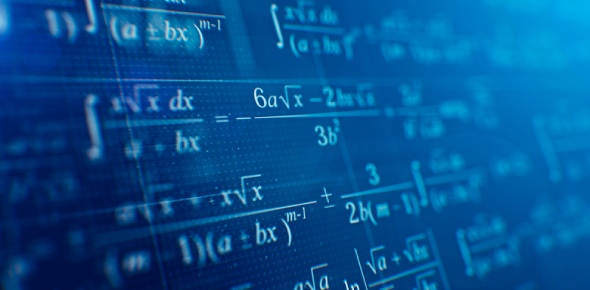# Mathematics - Chapter 3/ Figures And Lines

10 Questions | Total Attempts: 143SettingsMathematics - Chapter 3/ Figures and LinesFinish this test and enjoy it! You can do it.

Related Topics
• 1.
What is the name of this figure?
• A.

Square

• B.

Sphere

• C.

Cube

• D.

Rectangular prism

• 2.
What is the name of this figure?
• A.

Sphere

• B.

Circle

• C.

Sun

• D.

Cube

• 3.
The _______________________ are straight, intersecting lines.
• 4.
What is the name of this line?
• A.

Line

• B.

Line segment

• C.

Intersecting lines

• D.

Perpendicular lines

• 5.
What is the name of this figure?
• A.

Triangle

• B.

Square

• C.

Square pyramid

• D.

Point

• 6.
These lines never intersect. They are called ____________________.
• 7.
What is the name of this figure?
• A.

Rectangular prism

• B.

Cube

• C.

Ruler

• D.

Rectangle

• 8.
What is the name of the point northwest from Point C?
• A.

Point D

• B.

Point A

• C.

Point E

• D.

Point B

• 9.
An _________________________ is a line that crosses another line and never stops.
• 10.
What is the name of this figure?
• A.

Sphere

• B.

Cone

• C.

Cylinder

• D.

Cube